# PSAT Math : Drawing Conclusions from Graphs & Tables

## Example Questions

← Previous 1

### Example Question #1 : Drawing Conclusions From Graphs & Tables

Matt conducted a statistical experiment to determine the relationship between yearly salary earned and age. In this study, he assigned age (in years) as the independent variable, and yearly salary as the dependent variable. He drew a line of best fit and found a slope of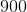. What does this mean?

Each month, a person's salary decreases by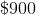Each month, a person's salary increases byEach year, a person's salary decreases byEach year, a person's salary increases byEach year, a person's salary increases byExplanation:

The slope of a line is the rate that a line increases or decreases. The question tells us that Matt looked at the relationship between age, in years; thus, the correct answer should include "each year", which eliminates the answer choices that include "each month". Finally the slope is, which is a positive number; thus, the line increases byeach year. This means that the correct answer is "Each year, a person's salary increases by".

### Example Question #1 : Drawing Conclusions From Graphs & Tables

Mrs. Frame conducted a statistical experiment to determine the relationship between test grades and the number of hours her students spent studying. In this study, she assigned the number of hours spent studying as the independent variable, and the test grades (in percentages) were assigned as the dependent variable. She plotted the data on a scatter plot and drew a line of best fit. If the slope of the best fit line wasand one of her data points was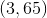, can we determine the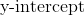of the best fit line? If yes, determine the.

No, thecan't be determined.

Yes ,thecan be determined: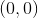Yes, thecan be determined: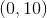Yes ,thecan be determined: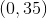Yes ,thecan be determined:Explanation:

The equation of the best fit line will be in slope intercept form:The question tells us that the slope isand we are provided with a data point, so we can plug in the known values into the equation to solve for the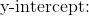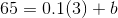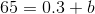We had to convert our percentage into a decimal in order to multiple, but we need to change it back to a percent before we subtract so that we arrive at the correct answer becauseis a percent based on the information from the question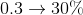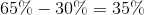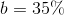which means the y-intercept is### Example Question #3 : Drawing Conclusions From Graphs & Tables

Mr. Miller conducted a statistical experiment to determine the relationship between final grades and the number of school days that his students missed. In this study, he assigned the number of missed school days as the independent variable, and the final grade was assigned as the dependent variable. He plotted his results on a scatter plot. If the results follow a linear relationship, what is a reasonable conclusion that could be found based these results?

A slope ofA negative slope

A positive slope

An undefined slope

A negative slope

Explanation:

We know, from attending school ourselves, that every day we learn something new in school. When a day of school is missed, there is a lot of catch up that needs to be done, but the teacher's instructions and lesson given each day can't be repeated the day you return, because the teacher has to move on with the rest of the class. If you missed a week of school, that's five days of lessons that were missed. Wouldn't it be challenging to catch up on what was missed, as well as learning what the teacher is currently teaching when you return? This would likely be challenging; thus, we can conclude that the more days of school missed, the lower a students final grade will be. As the number of days missed increases, the final grade will decrease; thus, the best fit line will have a negative slope.

### Example Question #4 : Drawing Conclusions From Graphs & Tables

Matt conducted a statistical experiment to determine the relationship between yearly salary earned and age. In this study, he assigned age (in years) as the independent variable, and yearly salary as the dependent variable.  He plotted his results on a scatter plot. If the results follow a linear relationship, what is a reasonable conclusion that could be found based these results?

A slope ofAn undefined slope

A positive slope

A negative slope

A positive slope

Explanation:

If you were to get a part time job when you turn, it's likely that you'll make minimum wage because it's your first job and you haven't finished high school, nor would you have a college education. However, if you think about a doctor, graduating from medical school when he/she is aboutyears old, the doctor is likely going to make a lot more than minimum wage because he's gone through high school, college, and medical school. As you can probably assume, doctors make a salary much higher than minimum wage. Based on this scenario, we can conclude that as age increases, salary increases; thus, the slope of the best fit line will have a positive slope.

### Example Question #1 : Drawing Conclusions From Graphs & Tables

A school principal conducted a statistical experiment to determine the relationship between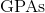and the number of hours spent studying each week. In this study, the principal assigned the number of hours spent studying as the independent variable, and the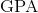was assigned as the dependent variable. He plotted his results on a scatter plot. If the results follow a linear relationship, what is a reasonable conclusion that could be found based these results?

A negative slope

An undefined slope

A slope ofA positive slope

A positive slope

Explanation:

We know, from attending school ourselves, that completing homework assignments and studying for quizzes and tests means that we will do better in school than if we didn't do those things. Completing homework and studying for tests takes time outside of school. Normally, the harder you study and the more time you spend studying, the more likely you are to do well in school. If you don't study at all, nor spend anytime completing homework assignments, your school grade will likely be lower than if you had spent time preparing and completing assignments; thus, we can conclude that the more time we spend studying, the higher ourwill be. As the number of hours studied increases, thewill increase; thus, the best fit line will have a positive slope.

### Example Question #1 : Use The Equation Of A Linear Model To Solve Problems: Ccss.Math.Content.8.Sp.A.3

Mrs. Frame conducted a statistical experiment to determine the relationship between test grades and the number of hours her students spent studying. In this study, she assigned the number of hours spent studying as the independent variable, and the test grades (in percentages) were assigned as the dependent variable. She plotted her results on a scatter plot. If the results follow a linear relationship, what is a reasonable conclusion that could be found based these results?

An undefined slope

A slope ofA positive slope

A negative slope

A positive slope

Explanation:

We know, from attending school ourselves, that when we have a test coming up that we want to do well on, we'll study for the test. Normally, the harder you study and the more time you spend studying, the more likely you are to do well on the test. If you don't study at all, and don't know the material that's being covered on a test, you'll like do poorly on a test; thus, we can conclude that the more time we spend studying, the higher our test grade will be. As the number of hours studied increases, the score on the test will increase; thus, the best fit line will have a positive slope.

### Example Question #7 : Drawing Conclusions From Graphs & Tables

A doctor conducted a statistical experiment to determine the relationship between age and height. In this study, she assigned age as the independent variable, and height (in inches) as the dependent variable. She plotted the data on a scatter plot. The doctor drew a line of best fit and found theto be. What does this mean?

A newborn baby will have an average height of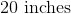The average person has a height ofEvery year a person grows byEvery month a person grows byA newborn baby will have an average height ofExplanation:

The question tells us that age is the independent variable, or the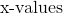, and height is the dependent variable, or the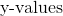Theis when; thus, when a person is just born their age isand their average height is### Example Question #1 : Use The Equation Of A Linear Model To Solve Problems: Ccss.Math.Content.8.Sp.A.3

A used car dealership conducted a statistical experiment to determine the relationship between the age of a car and the cost. In this study, he assigned age as the independent variable, and price as the dependent variable. He plotted the data on a scatter plot and drew a line of best fit. Thewas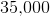. What does this mean?

The price of a brand new car is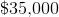AS a car ages, the price decreases byAs a car ages, the price increases byThe slope for the best fit line of this data set isThe price of a brand new car isExplanation:

The question tells us that age is the independent variable, or the, and price is the dependent variable, or theTheis when; thus, when a car is brand new its age isand the average price is### Example Question #9 : Drawing Conclusions From Graphs & Tables

A used car dealership conducted a statistical experiment to determine the relationship between the age of a car and the cost. In this study, he assigned age as the independent variable, and price as the dependent variable. He plotted the data on a scatter plot and drew a line of best fit.  If the results follow a linear relationship, what is a reasonable conclusion that could be found based these results?

A negative slope

A slope ofAn undefined slope

A positive slope

A negative slope

Explanation:

Imagine that you were buying a car. You have two options: a brand new car for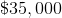or the same car, but a ten year older model for, which one would you pick? Most likely, you would take the brand new car because they are the same price. As a car get's older, it decreases in value because it becomes outdated and it the car will likely have been driven a lot more miles the older it gets. This means that the slope of the best fit line will be negative, since the price will decrease as the age increases.

### Example Question #10 : Drawing Conclusions From Graphs & Tables

A doctor conducted a statistical experiment to determine the relationship between weight and height. In this study, she assigned weight as the independent variable, and height (in inches) as the dependent variable. She plotted the data on a scatter plot.  If the results follow a linear relationship, what is a reasonable conclusion that could be found based these results?

A negative slope

A slope ofA positive slope

An undefined slope